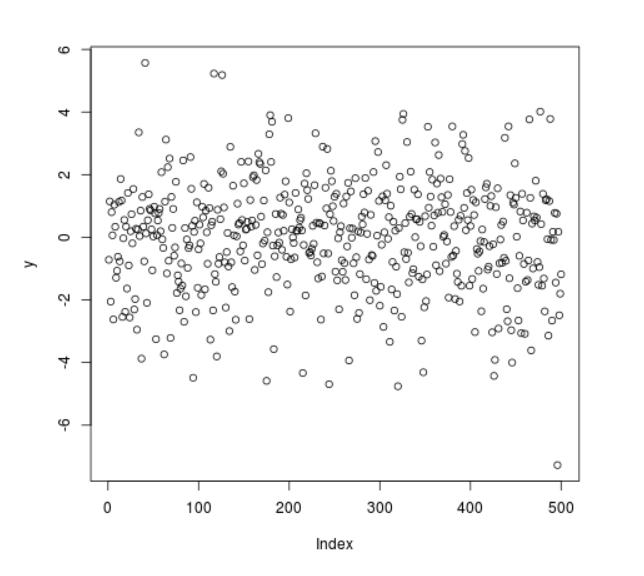Skip to content
Related Articles
Compute Randomly Drawn Logistic Density in R Programming – rlogis() Function
• Last Updated : 25 Jun, 2020

`rlogis()` function in R Language is used to compute random logistic density among a range of inputs.

Syntax: rlogis(N)

Parameters:
N: Sample Size

Example 1:

 `# R program to generate ``# random logistic density points`` ` `# Setting seed for``# random number generation``set``.seed(``1000``)`` ` `# Set sample size``N <``-` `20`` ` `# Calling rlogis() Function``y <``-` `rlogis(N)``y `

Output:

```  -0.71779506  1.14636578 -2.05114837  0.80365206  0.06563317 -2.62196720
  1.03929969  0.33730220 -1.29048105 -1.06622107 -0.61931410  1.12795649
 -0.76720400  1.86443303  1.17562774 -2.54319669 -0.02953117  0.55442681
 -2.37810163  0.33889069
```

Example 2:

 `# R program to generate ``# random logistic density points`` ` `# Setting seed for``# random number generation``set``.seed(``1000``)`` ` `# Set sample size``N <``-` `500`` ` `# Calling rlogis() Function``y <``-` `rlogis(N)`` ` `# Plot a graph``plot(y)`

Output:My Personal Notes arrow_drop_up